# DAV Class 8 Maths Chapter 10 Worksheet 1 Solutions

The DAV Maths Class 8 Solutions and DAV Class 8 Maths Chapter 10 Worksheet 1 Solutions of Parallel Lines offer comprehensive answers to textbook questions.

## DAV Class 8 Maths Ch 10 WS 1 Solutions

Question 1.
In the given figure, l ∥ m ∥ n and p is a transversal. If ∠1 = 110°, find angles x, y, z and w.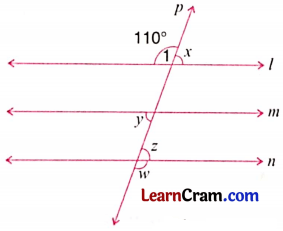Given that l ∥ m ∥ n and p is the transversal.
∴ ∠1 + ∠x = 180° [Supplementary angles]
⇒ 110° + ∠x = 180°
⇒ ∠x = ∠y [Corresponding angles]
70° = ∠y
∠y = 70°
∠y = ∠z [Alternate angles]
70° = ∠z
Hence, ∠z = 70°

Question 2.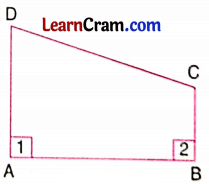∠1 = 90°
∠2 = 90°
AB is a transversal.
∴∠1 + ∠2 = 180°.
∴ ∠1 and ∠2 are supplementary angles.
Hence, AB ∥ CD.Question 3.
Draw a line segment $$\overline{\mathrm{AB}}$$ = 6 cm. Mark two points P and Q on it. Draw lines perpendicular to AB through P and Q (PR and QS). What can you say about PR and QS? Are these parallel? Justify your answer.
In the given figure,PR ⊥ AB and QS ⊥ AB
∴ ∠1 = ∠2 = 90°
But ∠1 and ∠2 are correspondings angles
∴ $$\overleftrightarrow{\mathrm{PR}} \| \overrightarrow{\mathrm{QS}}$$

Question 4.
In the given figure, l∥m∥n. Find ∠x and ∠y.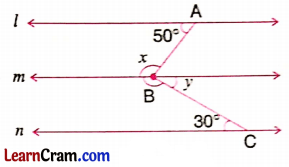l ∥ m ∥ n (given)
∠x + 50° = 180° [Sum of interior angles]
⇒ ∠x = 180° – 50°
∠x = 130°
∠y = 30° [Pair of alternate angles]

Question 5.
ABCD is a quadrilateral in which all the four angles are equal. Show that AB∥CD and AD ∥BC.
Let the measure of each angle of the quadrilateral ABCD be x.
Then ∠A = ∠B = ∠C = ∠D = x.We know that,
the sum of all the angles of a quadrilateral = 360°
⇒ ∠A + ∠B + ∠C + ∠D = 360°
⇒ x + x + x + x = 360°
⇒ 4x = 360°
⇒ x = 90°
So, ∠A = ∠B = ∠C = ∠D = 90°
Now, ∠A + ∠B = 90° + 90° = 180°.
They form a pair of interior angles on the same side of a transversal.
Hence, $$\overline{\mathrm{AD}} \| \overline{\mathrm{BC}}$$.
Similarly, ∠B + ∠C = 90° + 90° = 180°.
∴ They form a pair of interior angles on the same side of a transversal.
Hence, $$\overline{\mathrm{AB}} \| \overline{\mathrm{DC}}$$.

Question 6.
In the given figure, if ∠BAO = ∠DCO and OC = OD, show that $$\overline{\mathrm{AB}} \| \overline{\mathrm{CD}}$$.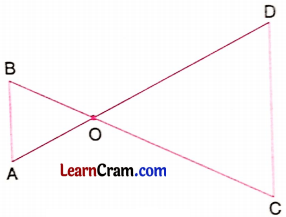Given that ∠BAO = ∠DCO .. .(i)
and OC = OD …..(ii)
Consider ΔOCD.
Since OC = OD
⇒ ∠DCO = ∠CDO (Angles opposite to equal sides of a triangle are equal)
⇒ ∠BAO = ∠CDO [From (i]
But these are alternate angles.
∴ AB ∥ CD.

Question 7.
In the given figure, ∠A =75° and $$\overline{\mathrm{CE}} \| \overline{\mathrm{AB}}$$. If ∠ECD = 40°, find the other two angles of the triangle ABC.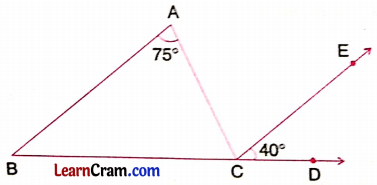$$\overline{\mathrm{AB}} \| \overline{\mathrm{CE}}$$ [Given]
∠BAC = ∠ACE
75° = ∠ACE
∠ACD = ∠ACE + ∠ECD
= 75° + 40° = 115°
∠ACB + ∠ACD = 180° [Corresponding angles]
∠ACB + 115° = 180°
∠ACB = 180° – 115° = 65°
∠ABC = ∠ECD [Corresponding angles]
∴ ∠ABC = 40°
Hence, ∠ACB = 65° and ∠ABC = 40°.

Question 8.
In the given figure, l∥ m, find ∠x, ∠y and ∠z.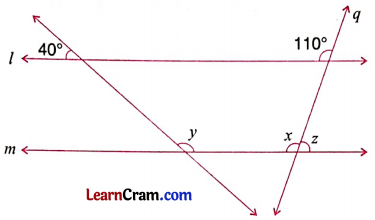l ∥ m (given)
and q is transversal.
∴ ∠x = 110° [Corresponding angles]
∠x + ∠z = 180° (Supplementary angles)
110° + ∠z = 180°
∴ ∠z = 180° – 110° = 70°
40° + ∠1 = 180°
∠1 = 180° – 40° = 140°
But ∠1 = ∠y (Alternate angles)
∴ ∠y = 140°
Hence, ∠x = 110°, ∠y = 140° and ∠z = 70°.Question 9.
In the given figure, show that: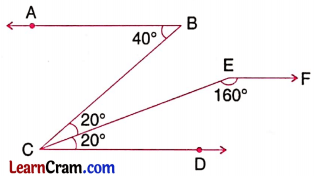(i) $$\overline{\mathrm{AB}} \| \overline{\mathrm{CD}}$$
∠ABC = 40° (Given)
∠BCD = 20° + 20° = 40° (Given)
∴ ∠ABC = ∠BCD
But they are alternate angles.
∴ $$\overline{\mathrm{AB}} \| \overline{\mathrm{CD}}$$

(ii) $$\overline{\mathrm{CD}} \| \overline{\mathrm{EF}}$$
∠ECD = 20° (Given)
∠CEF = 160° (Given)
∠ECD + ∠CEF = 20° + 160°
= 180°
∴ They are interior angles on the same side of transversal CE.
∴ $$\overline{\mathrm{CD}} \| \overline{\mathrm{EF}}$$

(iii) $$\overline{\mathrm{AB}} \| \overline{\mathrm{EF}}$$
$$\overline{\mathrm{AB}} \| \overline{\mathrm{CD}}$$ [from (i)]
$$\overline{\mathrm{AB}} \| \overline{\mathrm{CD}}$$ [from (ii)]
∴ $$\overline{\mathrm{AB}} \| \overline{\mathrm{CD}}$$
Hence proved.### DAV Class 8 Maths Chapter 10 Worksheet 1 Notes

If two lines in a two dimensional plane do not intersect each other, they are called parallel lines.

Transversal line:
Any line which intersects the parallel lines is called transversal line.Here in the alongside figure, l || m and t is the transversal intersecting l and m at A and B respectively.

Some pair of angles:

1. Interior angles: ∠2 and ∠3, ∠6 and ∠7
2. Pair of alternate angles: ∠2 and ∠7, ∠3 and ∠6
3. Pair of vertically opposite angles: ∠1 and ∠6, ∠2 and ∠5, ∠3 and ∠8, ∠4 and ∠7.
4. Pair of corresponding angles: ∠1 and ∠3, ∠5 and ∠7, ∠2 and ∠4, ∠6 and ∠8.

Supplementary angles:
Any two angles whose sum is 180° are called supplementary angles.Example 1.
In the given figure, ∠2 = 65°, find ∠1, ∠4 and ∠3.Solution:
(i) ∠1 + ∠2 = 180° [Supplementary angles)
∠ + 65° = 180°
∴ ∠1 = 180° – 65° = 115

(ii) ∠1 = ∠4 [Corresponding angles]
115° = ∠4
∴ ∠4 = 115°

(iii) ∠3 + ∠4 = 180° [Supplementary angles]
∠3 + 115° = 180°
∠3 = 180° – 115°
= 65°Example 2.
Divide a given line AB = 4 cm into 5 equal parts.
Solution:Steps of Construction:
(i) Draw AB = 4 cm.
(ii) At A, draw a ray AP making an acute angle with $$\overline{\mathrm{AB}}$$.
(iii) At B, draw AP || BQ on opposite side.
(iv) With the help of compass, mark 5 points, A1, A2, A3, A4 and A5 on AP at equal distances and B1, B2, B3, B4 and B5 on BQ.
(v) Join A to B5 and B to A5.
(vi) Join A1B4, A2B3, A3B2 and A4B1.
(vii) Measure $$\overline{\mathrm{AC}_1}$$, $$\overline{\mathrm{C}_1 \mathrm{C}_2}$$, $$\overline{\mathrm{C}_2 \mathrm{C}_3}$$, $$\overline{\mathrm{C}_3 \mathrm{C}_4}$$ and $$\overline{\mathrm{C}_4 \mathrm{~B}}$$ which are equal.